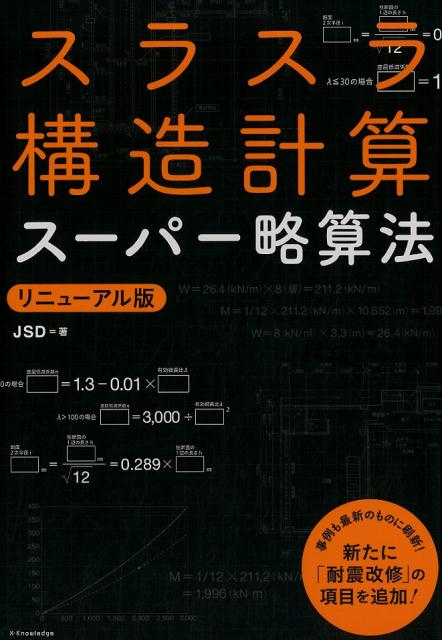PR

# Frame structure | strength calculation

A super overview of the strength calculation of the frame strcuture.

Specialized strength calculations are performed when designing a building, but detailed calculations are almost never performed at the frame level.

If you select the same structure and materials as the existing one, it will be OK for the time being.

Even if you try to reduce the number of parts with strange strength calculations, if there is no cost advantage and you are in a dangerous situation, let’s go up one size.

This kind of thinking saves design effort.

However, as an engineer, I feel that it is a waste if I do not know the outline of the calculation method.

Knowing what kind of idea is being designed will help you to respond flexibly.

## model

The model we are considering is as follows.

This is the so-called trestle.

A tank, a reactor, etc. are set in the center with four legs, and the load of the equipment is received by the horizontal ribs of the frame.

Equipment is supported by brackets.

Let W be the weight of the equipment.

## beam strength calculation

The first step in the trestle strength calculation is the beam strength calculation.

The stilt beam of member A applies the basic formula of material mechanics itself.

Support both ends and calculate the deflection of the beam with W/4 in the middle of the beam (because there are four stilt beams).

Now select the members so that the deflection/bending stress of the beam is below the allowable value.

We will increase the second moment of area.

The load received by member A is transmitted to member B as it is.

Member B also performs the same beam deflection calculation as member A.

The position where the load is applied is slightly different from that of member A, so some caution is required.

Calculate the size of member B in the same way as member A, but since member B is generally longer , a larger member is required.

The vertical load received by member B is transmitted to member C, which is the column part of the frame.

For member C, perform buckling calculations.

This is also the basis of mechanics of materials.

Seismic load must be taken into account when calculating strength.

Consider the seismic load as the load to receive the horizontal movement of equipment due to an earthquake.

The seismic load itself depends on the coefficient.

That’s the idea.

The seismic coefficient varies depending on the region and calculation standards.

Two loads, a horizontal load and a vertical load, are generated on member C by an earthquake.

Vertical load: Moment that resists the seismic load of equipment and the moment generated by the difference in the center of gravity.

Horizontal loads are easy to understand.

Vertical loads can be a little confusing.

(Center of gravity of equipment – height of members A and B of frame) x (earthquake load) = (length of member B) x (vertical load)

That’s the calculation.

This is the reason why it is better if the center of gravity of the tank and the bracket position are close.

The left-hand side becomes almost zero, and the need to consider the vertical load disappears.

Vertical loads are also assessed by performing beam deflection calculations.

For member C, consider buckling due to equipment load and deflection due to seismic load , and select a member that satisfies both.

In the case of a rotating device such as an agitator, the force due to agitation is considered as a horizontal load. This is also a simple calculation with a coefficient in the same way as the seismic load. This is because it changes depending on the shape of the stirrer, the presence or absence of accessories, and the contents.

For professional rotating equipment, we ask the manufacturer to calculate the load data. Calculation of dynamic loads as well as simple static loads is required.

If a diagonal member is attached, it will be in the direction of relieving the horizontal and vertical loads of member C.

You can think of it like the image below.

## Mounting bolts

Mounting bolts are calculated from the force and moment applied to member C.

The idea is almost the same as the calculation for anchor bolts.

Note that the stress on the beam is a composite calculation of horizontal and vertical stress.

## comprehension using mathematics

While saying a rough understanding, I will also show an understanding using formulas at the end.

The following conditions are commonly used.

M: bending moment

σ: bending stress

Z: section modulus

Subscripts A, B, C … indicate A, B, C of members

### Material A

Calculate the bending stress of the beam of member A.

Use the formula for load W/4, length LA and supports on both ends.

$$M_A=\frac{W}{4}\frac{L_A}{2}\frac{1}{2} = \frac{WL_A}{16}$$

$$σ_A=\frac{M_A}{Z_A} = \frac{WL_A}{16Z_A}$$

### Material B

Calculate member B in the same way as member A.

$$M_B=\frac{W}{8}\frac{L_B-L’_B}{2}= \frac{W(L_B-L’_B)}{16}$$

$$σ_B=\frac{M_B}{Z_B} =\frac{W(L_B-L’_B)}{16Z_B}$$

### Material C

Let’s take a look at component C.

$$F=\frac{1}{2}F_0$$

On the other hand, since it receives the moment due to the height difference, it also receives the vertical load. Let this be F’,

$$F’\frac{L_B}{2} = F_0(h-L_C)$$

$$F’=\frac{h-L_C}{L_B}F$$

### Material D

Let’s also consider the case where there is a component D.

As you can see, the formula becomes a little more complicated when diagonal member D is included.

Considering the force balance (positive and negative signs are attached appropriately), the vertical and horizontal directions are as follows.

$$F_D\frac{L_C}{L_D}+F_C-F’=0$$

$$F_D\frac{L_B}{L_D}-F=0$$

From these formulas:

$$F_D=F\frac{L_D}{L_B}$$

$$R_A=F’=\frac{h-L_C}{L_B}F$$

If the cross-sectional area of ​​member C is A, the tensile stress and shear stress applied to the fixing point of member C are respectively,

$$σ_k=\frac{R_A}{A}$$

$$τ_k=\frac{F}{A}$$

When calculating the principal stress σm from these,

$$σ_m=\frac{1}{2}σ_k+\frac{1}{2}\sqrt{{σ_k}^2+4{τ_k}^2}$$

## referenceスラスラ構造計算スーパー略算法リニューアル版 [ JSD ]created by Rinker
¥3,080(2023/09/29 14:12:43時点 楽天市場調べ-詳細)

## lastly

I explained the image of the strength calculation of the frame.

The beam deflection calculation of material mechanics is the basis.

We will add horizontal loads such as buckling calculations and earthquakes and agitation to this.

It’s getting less and less to do detailed calculations, but I’d like to understand the concept.